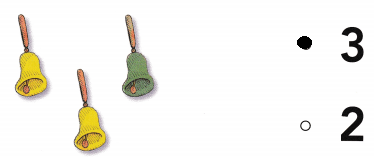# Texas Go Math Kindergarten Lesson 9.2 Answer Key Compose Numbers 9 and 5

Refer to our Texas Go Math Kindergarten Answer Key Pdf to score good marks in the exams. Test yourself by practicing the problems from Texas Go Math Kindergarten Lesson 9.2 Answer Key Compose Numbers 9 and 5.

## Texas Go Math Kindergarten Lesson 9.2 Answer Key Compose Numbers 9 and 5

Explore

DIRECTIONS: Place red and yellow counters in the five frame as shown. Trace the number that shows how many counters in all. Write the number of yellow counters. Trace the symbol. Write the number of red counters.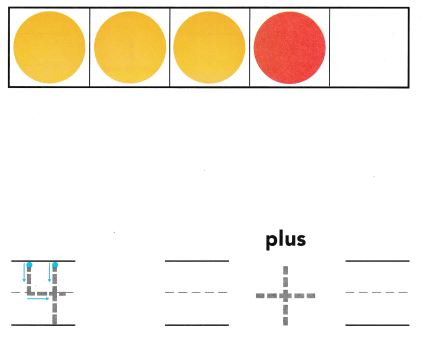Explanation:
The number of red counters are 1
the number of yellow counters are 3
Total number of counters 4
3 + 1 = 4

Share and Show

DIRECTIONS: 1-2. Place counters on the five frames as shown. Write the number that shows how many counters in all. Write the number of yellow counters. Trace the symbol. Write the number of red counters.

Question 1.Explanation:
The number of red counters are 1
the number of yellow counters are 4
Total number of counters 5
4 + 1 = 5

Question 2.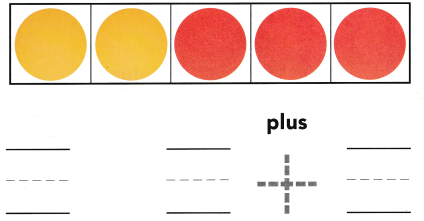Explanation:
The number of red counters are 3
the number of yellow counters are 2
Total number of counters 5
3 + 2 = 5

DIRECTIONS: 3-4. Place counters on the five frames as shown. Write the number that shows how many counters in all. Write the number of yellow counters. Trace the symbol. Write the number of red counters.

Question 3.Explanation:
The number of red counters are 2
the number of yellow counters are 2
Total number of counters 4
2 + 2 = 4

Question 4.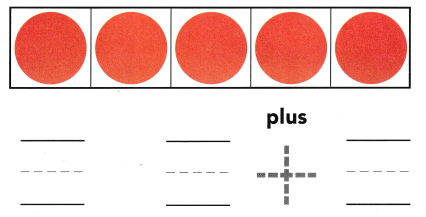Explanation:
The number of red counters are 5
the number of yellow counters are 0
Total number of counters 5
5 = 5 + 0

HOME ACTIVITY • Show your child two sets of two objects. Have him or her put the sets together and tell how many in all.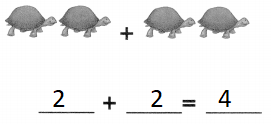Explanation:
There are 2 sets of 2 turtles
2 + 2 = 4
so, there are 4 turtles

DIRECTIONS: 5. Five apples are on the table. Three apples are red and two apples are green. Write the number to show how many apples in all. Write the numbers and trace the symbol to show the apples being put together. 6. Choose the correct answer. How many toy animals are there?

Problem Solving

Question 5.Explanation:
Five apples are on the table. Three apples are red and two apples are green
3 + 2 = 5

Question 6.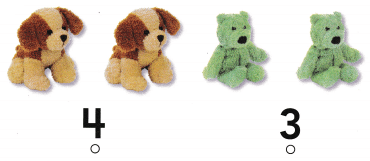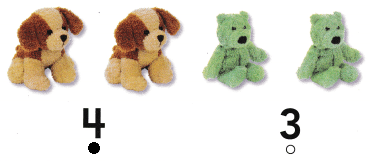Explanation:
The total number of teddy bears  are 4

### Texas Go Math Kindergarten Lesson 9.2 Homework and Practice Answer Key

DIRECTIONS: 1-2. Write the number that shows how many counters in all. Write the number of red counters. Trace the symbol. Write the number of yellow counters.

Question 1.Explanation:
The number of red counters are 3
the number of yellow counters are 2
Total number of counters 5
5 = 3 + 2

Question 2.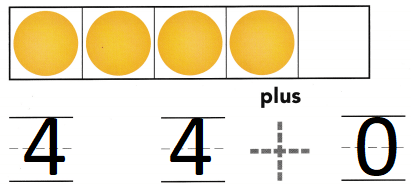Explanation:
The number of red counters are 0
the number of yellow counters are 4
Total number of counters 4
4 = 4 + 0

DIRECTIONS: Choose the correct answer. 3. How many drums are there? 4. How many bells ore there? 5. How many guitars are there?

Lesson Check

Question 3.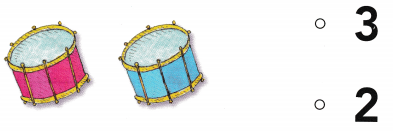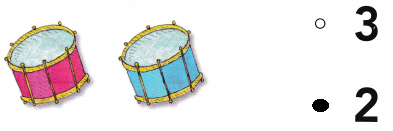Explanation:
The total number of drums are 2.

Question 4.